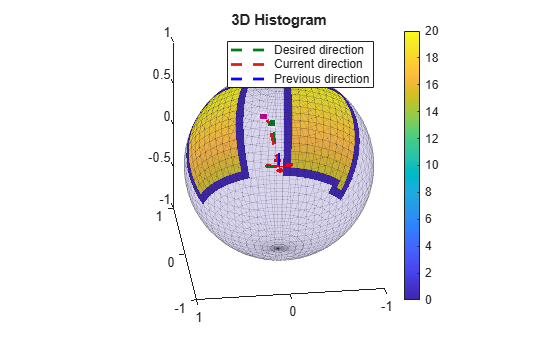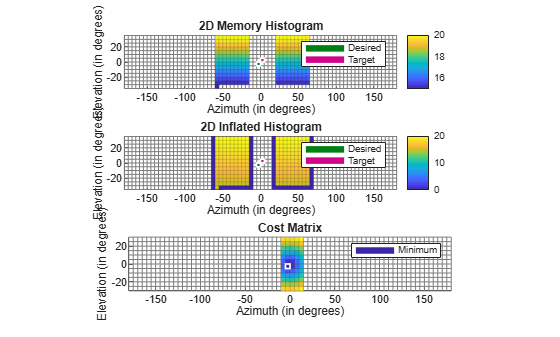# show

Display 3D vector field histogram

Since R2022b

## Syntax

``show(vfh3D)``
``show(vfh3D,Name=Value)``
``ax = show(___)``

## Description

````show(vfh3D)` displays the 3D histogram of the desired direction calculated by the 3DVFH+ algorithm. The figure also includes the UAV position, UAV orientation, obstacle positions, and target position specified to the `controllerVFH3D` object `vfh3D` at the most recent object call.```

example

````show(vfh3D,Name=Value)` specifies options using one or more name-value arguments. For example, `show(vfh3D,PlotsToShow="Cost Matrix")` displays only the cost matrix plot instead of the default histogram.```
````ax = show(___)` returns the axes handles of all displayed histograms, in addition to any combination of input arguments from previous syntaxes.```

## Examples

collapse all

Create a `controllerVFH3D` object.

`vfh3D = controllerVFH3D;`

Create obstacles.

```az = [-60:-20 20:60]*(pi/180); el = (-30:30)*(pi/180); [El,Az] = meshgrid(el,az);```

Specify the distances of the obstacles from the sensor, and convert to Cartesian coordinates.

```obstacleDist = linspace(15,20,numel(El(:))); [xSensor,ySensor,zSensor] = sph2cart(Az(:),El(:),obstacleDist');```

Align the sensor and histogram frames.

`vfh3D.SensorOrientation = [-180 0 0];`

Specify the sensor range limits.

`vfh3D.DistanceLimits = [0.2 25];`

Specify the current UAV position and orientation, the locations of obstacles, and the target position for the UAV.

```uavPosition = [0; 0; 0]; uavOrientation = [1; 0; 0; 0]; sensorPoints = [xSensor ySensor zSensor]; targetPosition = [20; 0; 0];```

Compute an obstacle-free direction and desired yaw for the UAV, and return the status of the obstacle-free direction.

```[desiredDirection,desiredYaw,status] = vfh3D(uavPosition, ... uavOrientation, ... sensorPoints, ... targetPosition);```

Visualize the default histogram of the calculated direction.

```show(vfh3D) axis equal```Visualize the 2D memory histogram, 2D inflated histogram, and cost matrix.

```figure ax(1) = subplot(3,1,1); ax(2) = subplot(3,1,2); ax(3) = subplot(3,1,3); show(vfh3D, ... Parent=ax, ... PlotsToShow=["2D Memory Histogram","2D Inflated Histogram","Cost Matrix"]) axis(ax,"equal") xlim(ax,"tight")```## Input Arguments

collapse all

3-D vector field histogram algorithm, specified as a `controllerVFH3D` object. This object contains properties for tuning the 3DVFH+ algorithm.

### Name-Value Arguments

Specify optional pairs of arguments as `Name1=Value1,...,NameN=ValueN`, where `Name` is the argument name and `Value` is the corresponding value. Name-value arguments must appear after other arguments, but the order of the pairs does not matter.

Example: `show(vfh3D,PlotsToShow="Cost Matrix")` shows the cost matrix plot.

Axes on which to plot the histograms, specified as an `Axes` object or an array of `Axes` objects.

Example: `Parent=ax`

Histogram plots to display, specified as a string scalar or string array. Each element must be specified as one of these options: `"3D Histogram"`, `"2D Memory Histogram"`, ```"2D Inflated Histogram"```, and `"Cost Matrix"` or `"All"`.

Example: `PlotsToShow="All"`

Example: `PlotsToShow="2D Memory Histogram"`

Example: ```PlotsToShow=["2D Memory Histogram","Cost Matrix"]```

Data Types: `string`

## Output Arguments

collapse all

Axes handles of the 3D vector field histograms, returned as an `Axes` object or an array of `Axes` objects.

## Version History

Introduced in R2022b